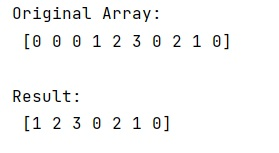# How to remove zeroes from the beginning of a NumPy array?

Given a NumPy array, we have to remove zeroes from the beginning of a NumPy array.
Submitted by Pranit Sharma, on March 01, 2023

NumPy is an abbreviated form of Numerical Python. It is used for different types of scientific operations in python. Numpy is a vast library in python which is used for almost every kind of scientific or mathematical operation. It is itself an array which is a collection of various methods and functions for processing the arrays.

## Removing zeroes from the beginning of a NumPy array

Suppose that we are given a NumPy array that contains some numeric values and there are some zero elements at the starting of this array. We need to find an efficient way to remove these zeroes from the beginning of this array.

For this purpose, we will use numpy.trim_zeroes() method which is used to trim the leading and/or trailing zeros from a 1-D array or sequence.

It takes an input array and a parameter trim which is of string type. A string 'f' represents trimming from the front and 'b' represents trimming from the back.

Let us understand with the help of an example,

## Python code to remove zeroes from the beginning of a NumPy array

```# Import numpy
import numpy as np

# Creating a numpy array
arr =  np.array([0, 0, 0, 1, 2, 3, 0, 2, 1, 0])

# Display original array
print("Original Array:\n",arr,"\n")

# Removing zeroes from beginning
res = np.trim_zeros(arr,'f')

# Display result
print("Result:\n",res,"\n")
```

Output:Languages: » C » C++ » C++ STL » Java » Data Structure » C#.Net » Android » Kotlin » SQL
Web Technologies: » PHP » Python » JavaScript » CSS » Ajax » Node.js » Web programming/HTML
Solved programs: » C » C++ » DS » Java » C#
Aptitude que. & ans.: » C » C++ » Java » DBMS
Interview que. & ans.: » C » Embedded C » Java » SEO » HR
CS Subjects: » CS Basics » O.S. » Networks » DBMS » Embedded Systems » Cloud Computing
» Machine learning » CS Organizations » Linux » DOS
More: » Articles » Puzzles » News/Updates